Home > A2C > Chapter 11 > Lesson 11.3.4 > Problem11-171

11-171.
1. If f (x) = x2 + 7x, calculate the values described below. Homework Help ✎

1. f (2)

2. f (−3)

3. f (i)

4. f (−3.5 + 1.5i)

5. Solve f (x) = 0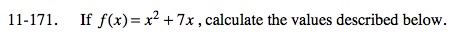For parts (a)-(d), substitute the value for x and simplify.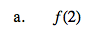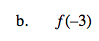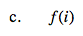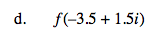x = −14.5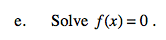Factor x2 + 7x = 0.

x = 0 and x = −7